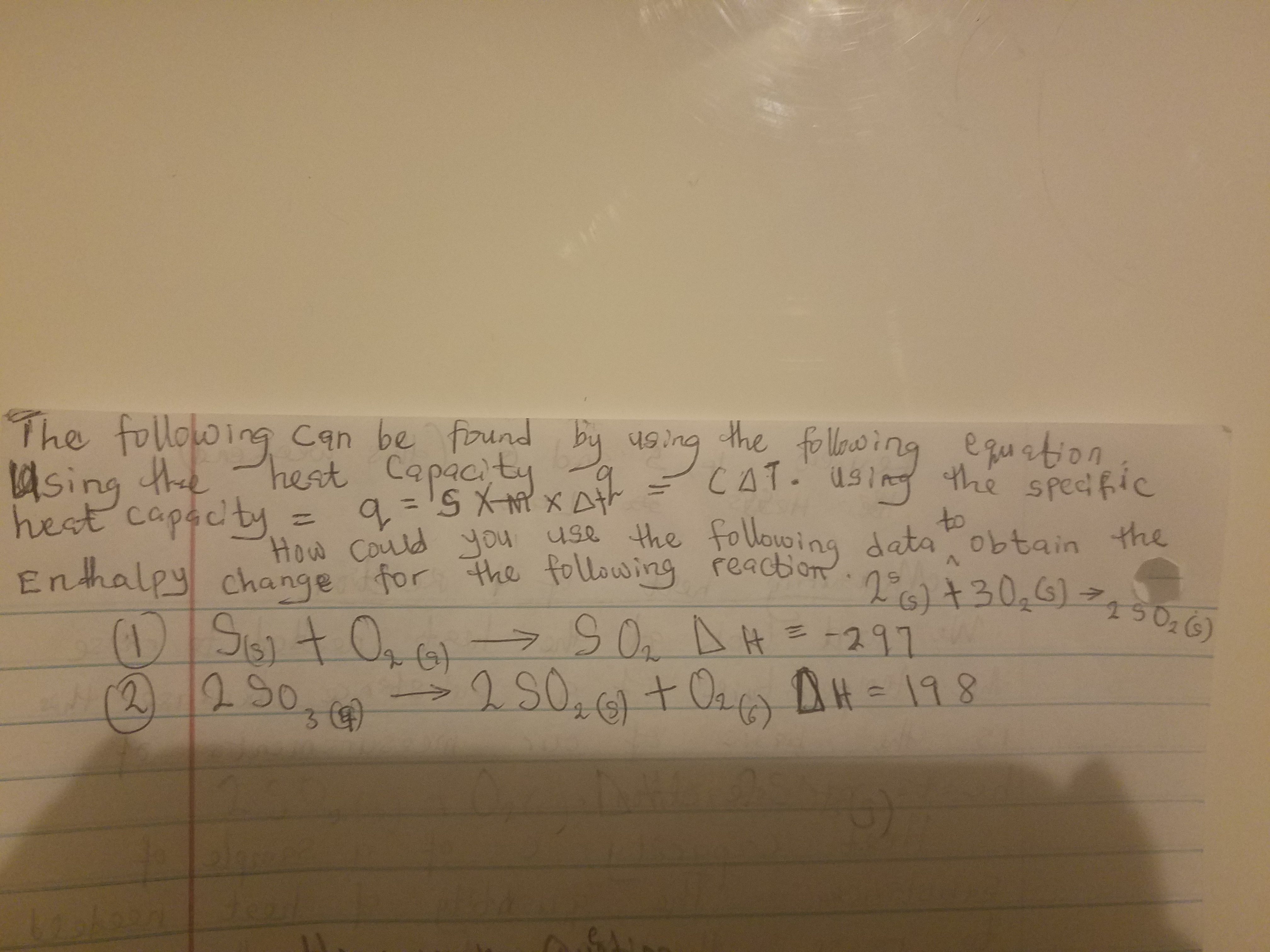# The following can be found by using the following equation: Using the heat capacity, q=cΔT Using the specific heat capacity, q = s×m×m×Δ How could you use the following data to obtain the enthalpy change for the following reaction: 2S(g) + 3O2(g)→2SO2(g) (1) S(g) + O2(g)→SO2 ΔH = -297 kJ/mol (2) 2 SO3(g)→2 SO2(g) + O2(g) ΔH = 198 So sorry, yes its heat (q) that is asked.